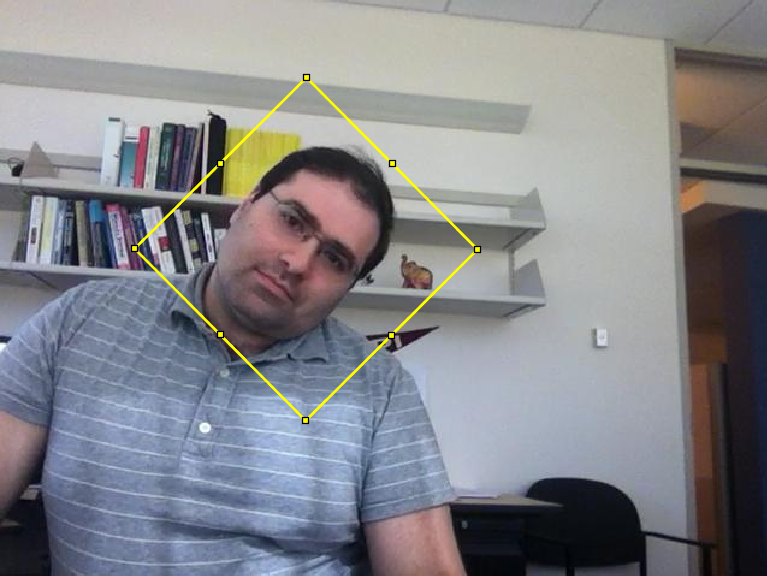# Minimally Bounded Rectangles, and more!

Brett‘s Pick this week is A suite of minimal bounding objects, by John D’Errico. I also give a shout-out to julien diener, for his 2D minimal bounding box function.

During the course of developing some code to automatically track objects in videos, I found that I needed to create a “minimal bounding rectangle” to enclose detected points. There is a very nice demo in the Computer Vision Toolbox in which points used to track a moving face are delineated by a polygon created by the polyshape command. All well and good; it works nicely, and is very fast. But for other reasons, I preferred to work with an ‘images.roi.Rectangle’ object, as created by the drawrectangle command (which is itself a convenience function for rectangle().)

A modified version of the aforementioned example looks like this:

vidObj = VideoReader('tilted_face.avi');
togglefig('Face Tracker')
imgH = imshow(videoFrame);
bbox = faceDetector(videoFrame);
bboxPoints = bbox2points(bbox(1, :));
thisPoly = polyshape(bboxPoints);
hold on
polyH = plot(thisPoly, 'FaceAlpha', 0, 'EdgeColor', 'y', 'LineWidth', 2);
points = detectMinEigenFeatures(rgb2gray(videoFrame), 'ROI', bbox);
pointTracker = vision.PointTracker('MaxBidirectionalError', 2);
points = points.Location;
initialize(pointTracker, points, videoFrame);
oldPoints = points;
tic;
while hasFrame(vidObj)
% Get the next frame, update the visualization:
imgH.CData = videoFrame;
% Track the points. Note that some points may be lost.
[points, isFound] = pointTracker(videoFrame);
visiblePoints = points(isFound, :);
oldInliers = oldPoints(isFound, :);
if size(visiblePoints, 1) >= 2 % need at least 2 points
[xform, oldInliers, visiblePoints] = estimateGeometricTransform(...
oldInliers, visiblePoints, 'similarity', 'MaxDistance', 4);
bboxPoints = transformPointsForward(xform, bboxPoints);
thisPoly = polyshape(bboxPoints);
polyH.Shape = thisPoly;
oldPoints = visiblePoints;
setPoints(pointTracker, oldPoints);
end
imgH.CData = videoFrame;
drawnow
end
t = toc;
% Clean up
release(pointTracker);

% (Processing the while loop takes about 6.5 seconds on my laptop.)But how would one replace the polygon with a rotated rectangle? Fortunately for us, there’s the File Exchange, where a search for “minimal bounding rectangle” quickly turned up John’s “suite” of functions for minimally bounding circles, spheres, parallelograms, quadrilaterals, semicircles, triangles, and rectangles.

As we’ve come to expect, John’s functions are well written and commented. It was a simple matter to replace the polygon with a minimally bounded rectangle, which not surprisingly computes the convex hull of the input points. One note: John’s function returned the x- and y- coordinates of the target rectangle, and the area and perimeter of the same:

[rectx,recty,area,perimeter] = minboundrect(x,y,metric)


(In fact, one can elect, using the ‘metric’ parameter, to minimize by area or perimeter, with area being the default.) I needed the rotation angle of the triangle, to use as an input in drawrectangle. It took a moment to modify minboundrect to return as an additional output the edgeangles parameter, which is computed internally. In moments, we had:

vidObj.CurrentTime = 0; % Reset and reuse the VideoReader object
togglefig('Face Tracker', true) %Clear and reuse figure
imgH = imshow(videoFrame);
bbox = faceDetector(videoFrame);
hRect = drawrectangle('Position', bbox, ...
'FaceAlpha', 0, 'Color', 'y', 'LineWidth', 2);
bboxPoints = bbox2points(bbox(1, :));
points = detectMinEigenFeatures(rgb2gray(videoFrame), 'ROI', bbox);
pointTracker = vision.PointTracker('MaxBidirectionalError', 2);
points = points.Location;
initialize(pointTracker, points, videoFrame);
oldPoints = points;
tic;
while hasFrame(vidObj)
% Get the next frame, update the visualization:
imgH.CData = videoFrame;
% Track the points. Note that some points may be lost.
[points, isFound] = pointTracker(videoFrame);
visiblePoints = points(isFound, :);
oldInliers = oldPoints(isFound, :);
if size(visiblePoints, 1) >= 2 % need at least 2 points
[xform, oldInliers, visiblePoints] = estimateGeometricTransform(...
oldInliers, visiblePoints, 'similarity', 'MaxDistance', 4);
bboxPoints = double(transformPointsForward(xform, bboxPoints));
% |vertToPos| is a helper function I wrote; it is the inverter for
% <https://www.mathworks.com/help/vision/ref/bbox2points.html |bbox2points|>.
test = 'john';
switch test
case 'john'
[rectx, recty, theta] = ...
minboundrect(bboxPoints(:,1), bboxPoints(:,2));
rectPos = vertToPos([rectx, recty]);
case 'julien'
[rect, theta] = minBoundingBox(bboxPoints');
rectPos = vertToPos(rect');
end
hRect.Position = rectPos;
hRect.RotationAngle = theta;
oldPoints = visiblePoints;
setPoints(pointTracker, oldPoints);
end
imgH.CData = videoFrame;
drawnow
end
t = toc;
% Clean up
release(pointTracker);